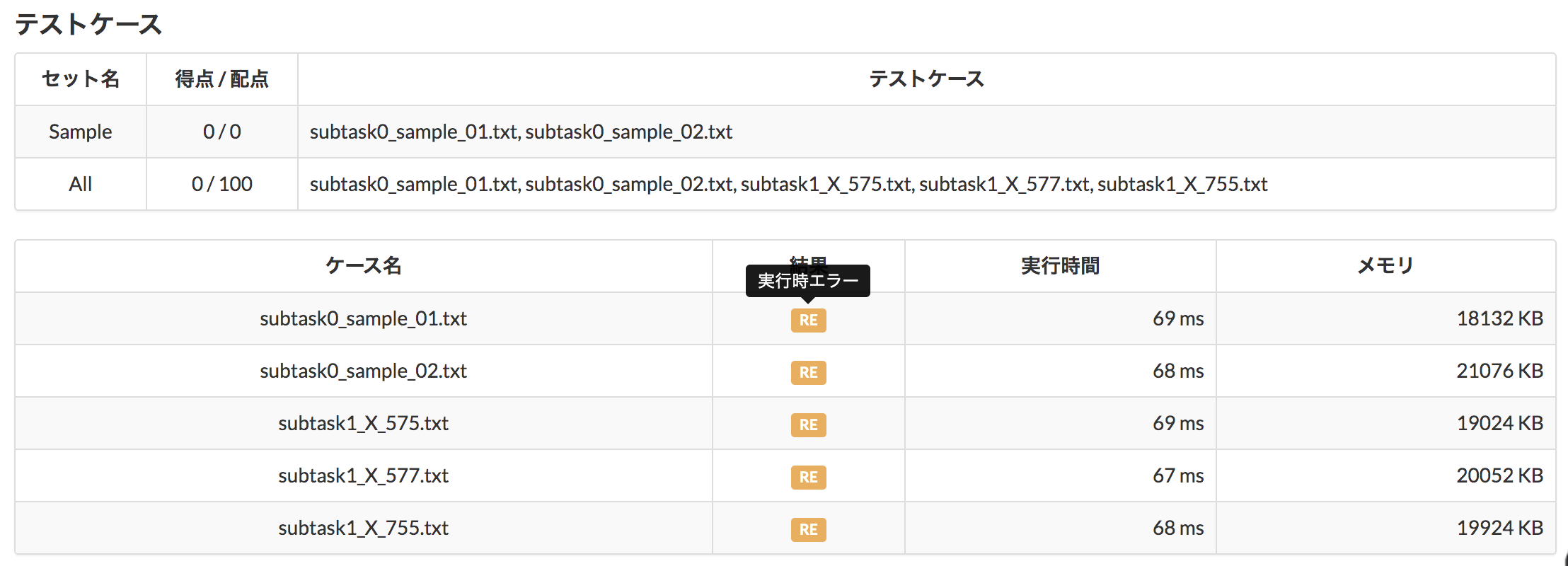# [JAVA] I immediately stumbled on the standard input method with AtCoder.

## Introduction

I've been working as an engineer until now, but I started doing competition pregramming because I thought it was difficult to implement because it was mainly in the upstream process. I managed to solve one question, but I will post a memorandum of what I stumbled upon.

This time I will write about the data entry `Scanner`.

## Challenged problem

Atcode's past score of 100 problem ... In other words, it's a simple problem.

## First submission

I managed to code while looking at various sites, and I was able to execute it from the vscode terminal and get the expected result. The following is the code content (probably a dirty code from an expert's point of view, but please forgive me ...)

``````public class Main {
public static void main(String args[]) {
String[] haiku = args;
int sum = 0;
for(int i = 0; i < 3; i++){
if(haiku[i].equals("7") || haiku[i].equals("5")){
sum = sum + Integer.valueOf(haiku[i]);
System.out.println(sum);
}
else{
}
System.out.println(haiku[i]);
}
if(sum != 17){
}
}
}
``````

## result

#### Run-time error ... I couldn't even check the contents ... \ (^ o ^) /## What went wrong

It seems that the input method was simply useless.

When I type `Java Main X X X` in the terminal, it's stored in the array ʻargs` separated by spaces, so I thought it was okay, but I was completely wrong.

## What should i do

It is recommended to use `Scanner` to get the input contents. Get the input at the `next ();` part and use it again to get the next input value.

``````Scanner sc = new Scanner(System.in);
int i = Integer.parseInt(sc.next());
``````

## 5th submission

I refactored the code many times (I'm sorry I want to use the term ...) and managed to clear it lol

``````import java.util.Scanner;
public class Main {
public static void main(String args[]) {
Scanner s = new Scanner(System.in);
int sum = 0;
for(int i = 0; i < 3; i++){
int haiku = Integer.parseInt(s.next());
if(haiku == 7 || haiku == 5 ){
sum = sum + haiku;
}
else{
}
}
if(sum != 17){